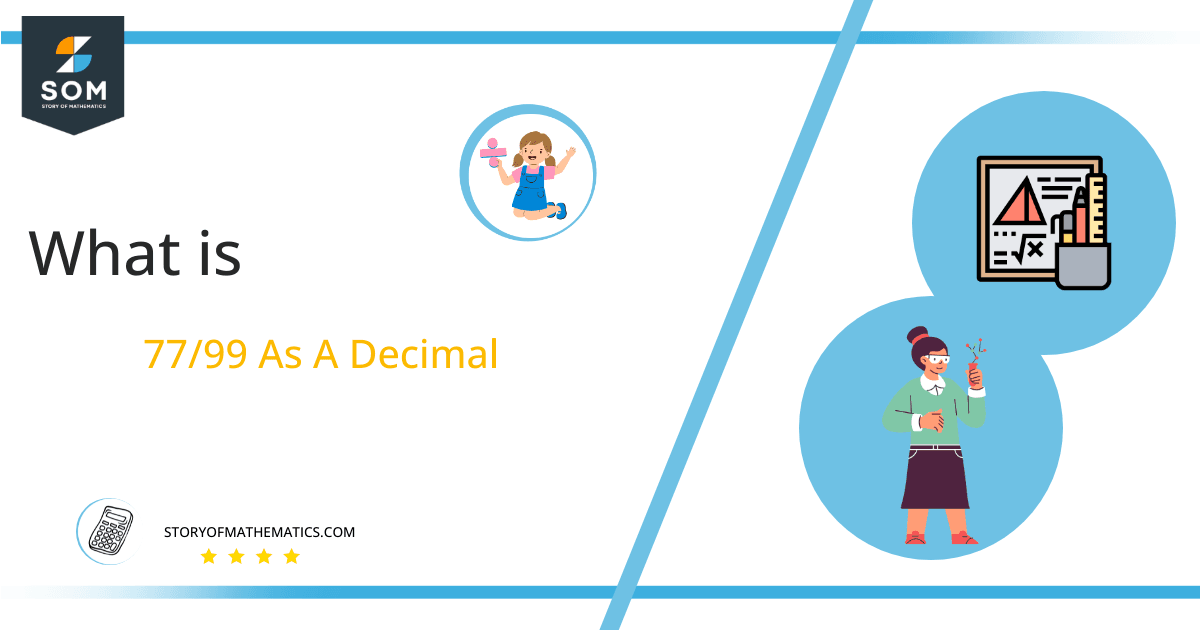# What Is 77/99 as a Decimal + Solution With Free Steps

The fraction 77/99 as a decimal is equal to 0.777.

In the fraction 77/99, 77 is the dividend, and 99 is the divisor. After applying the division method, the dividend will be divided into 99 equal parts. It is a non-terminating repeating decimal fraction.Here, we are more interested in the division types that result in a Decimal value, as this can be expressed as a Fraction. We see fractions as a way of showing two numbers having the operation of Division between them that result in a value that lies between two Integers.

Now, we introduce the method used to solve said fraction to decimal conversion, called Long Division, which we will discuss in detail moving forward. So, let’s go through the Solution of fraction 77/99.

## Solution

First, we convert the fraction components, i.e., the numerator and the denominator, and transform them into the division constituents, i.e., the Dividend and the Divisor, respectively.

This can be done as follows:

Dividend = 77

Divisor = 99

Now, we introduce the most important quantity in our division process: the Quotient. The value represents the Solution to our division and can be expressed as having the following relationship with the Division constituents:

Quotient = Dividend $\div$ Divisor = 77 $\div$ 99

This is when we go through the Long Division solution to our problem. The following figure shows the solution for fraction 77/99.Figure 1

## 77/99 Long Division Method

We start solving a problem using the Long Division Method by first taking apart the division’s components and comparing them. As we have 77 and 99, we can see how 77 is Smaller than 99, and to solve this division, we require that 77 be Bigger than 99.

This is done by multiplying the dividend by 10 and checking whether it is bigger than the divisor or not. If so, we calculate the Multiple of the divisor closest to the dividend and subtract it from the Dividend. This produces the Remainder, which we then use as the dividend later.

Now, we begin solving for our dividend 77, which after getting multiplied by 10 becomes 770.

We take this 770 and divide it by 99; this can be done as follows:

770 $\div$ 99 $\approx$ 7

Where:

99 x 7 = 693

This will lead to the generation of a Remainder equal to 770 – 693 = 77. Now this means we have to repeat the process by Converting the 77 into 770 and solving for that:

770 $\div$ 99 $\approx$ 7

Where:

99 x 7 = 693

This, therefore, produces another Remainder which is equal to 770 – 693 = 77. Now this means we have to repeat the process by Converting the 77 into 770 and solving for that:

770 $\div$ 99 $\approx$ 7

Where:

99 x 7 = 693

Finally, we have a Quotient generated after combining the three pieces of it as 0.777, with a Remainder equal to 77.Images/mathematical drawings are created with GeoGebra.